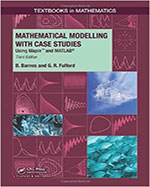Mathematical Modelling with Case Studies: Using Maple and MATLAB, Third Edition - Maplesoft Books - Maple Books, Maple Resources and Math BooksHome : Maplesoft Books : Mathematical Modelling with Case Studies: Using Maple and MATLAB, Third EditionDetails Search# Mathematical Modelling with Case Studies: Using Maple and MATLAB, Third Edition

## 2015

Description

Mathematical Modelling with Case Studies: Using Maple™ and MATLAB®, Third Edition provides students with hands-on modelling skills for a wide variety of problems involving differential equations that describe rates of change. While the book focuses on growth and decay processes, interacting populations, and heating/cooling problems, the mathematical techniques presented can be applied to many other areas.

The text carefully details the process of constructing a model, including the conversion of a seemingly complex problem into a much simpler one. It uses flow diagrams and word equations to aid in the model-building process and to develop the mathematical equations. Employing theoretical, graphical, and computational tools, the authors analyze the behavior of the models under changing conditions. The authors often examine a model numerically before solving it analytically. They also discuss the validation of the models and suggest extensions to the models with an emphasis on recognizing the strengths and limitations of each model.

The highly recommended second edition was praised for its lucid writing style and numerous real-world examples. With updated Maple™ code as well as new case studies and exercises, this third edition continues to give students a clear, practical understanding of the development and interpretation of mathematical models.

• Focuses on compartmental models, population models, and heat transfer problems
• Uses compartment diagrams and word equations to help readers conceptualize the formulation of differential equations
• Includes many case studies to provide a practical understanding of how the models are used in current research, such as environmental science, biology, and archaeology
• Supplies Maple codes in the text and online so results can be reproduced exactly
• Offers end-of-chapter problems for practicing with the formulation, analysis, and interpretation of differential equation models.  Solutions manual available for qualifying instructors.

MATLAB is a registered trademark of The MathWorks, Inc.

Categories:
Other Details
Language: English
ISBN: 978-1482247725
Publisher: CRC Press
 Do you know of a book that should be included in our list? Tell us about it!
 Title/Author: Category: Any Category Abstract Algebra Biology Calculus Chemistry Complex Analysis Computer Algebra Computer Science Conference Proceedings Courseware Cryptography Differential Equations Differential Geometry Dynamical Systems Economics Engineering Games Linear Algebra Maple Manuals Mathematical Modeling Number Theory Numerical Analysis Operations Research Optimization Physics Pre-Calculus Probability & Statistics Programming Recent Releases Vector Calculus Language: Any Language English French German Spanish Japanese Dutch Russian Swedish Polish Persian ChineseAbstract Algebra Biology Calculus Chemistry Complex Analysis Computer Algebra Computer Science Conference Proceedings Courseware Cryptography Differential Equations Differential Geometry Dynamical Systems Economics Engineering Games Linear Algebra Maple Manuals Mathematical Modeling Number Theory Numerical Analysis Operations Research Optimization Physics Pre-Calculus Probability & Statistics Programming Recent Releases Vector Calculus